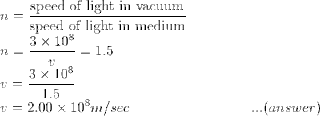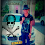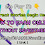## Numerical Problem on Light Reflection and Refraction

Q1: How fast does the light travel in a glass of refractive index 1.5?

By Snell's law, refractive index (n) is the ratio of i.e.

Q2:When a bright object is placed 10 cm away from a concave mirror, its real image is formed at a distance 40cm from the mirror. What is the focal length of the mirror?Q3: If the angle of incidence of a ray of light falling on the glass surface is 30° and the angle of refraction is 19°. what is the refractive index of glass?

Ans:
angle of incidence  i = 30°
angle of refraction  r = 19°
refractive index   n = sin i/sin r  = sin 30/sin 19
n = .5/.3256 = 1.535               ....(ans)

Q4: If a child crawls towards a mirror at a rate of 0.20 m/s, then at what speed will the child and her image come nearby to each other?

Ans: In 1 sec, the child moves towards the mirror by a distance of 0.20 meters. In the same second, her image also moves 0.20m closer.
Therefore, the child and her image comes closer to each other by 0.40m per second.

Q5: How many images will you see when two plane mirrors are perpendicular to each other?
Ans:The answer is three. You may see practically as shown in the figure. You may follow the link on multiple reflections to see more such images.

For two mirrors placed at any angle, the number of images formed by the mirrors can be determined by:

N = (360°/θ ) - 1

where θ is the angle between the mirrors and N is the number of images formed. For parallel mirrors θ = 0, hence N = ∞. (You may have seen this effect in barber shops).

Q6: A converging lens has a focal length of 15cm. An object is placed 60cm from the lens. Determine the image.

Ans:       Object Distance is u = -60cm
focal length f = 15 cm

Lens formula is:  1/v - 1/u = 1/f
1/v = 1/15 + (-1/60)
= 1/20
⇒            v = 20 cm
The image is real (+ve v) and is formed on the other side of the lens.
The magnification is:  m = 20/-60 = -1/3
The -ve sign of m tells that the image is inverted. The magnitude of m (magnification) is less than one, thus image formed is of reduced size.

Q7: What is the focal length of a lens that produces a real image three times as large as the object if the distance between image and object is 1.0m?

Ans:  magnification m = 3
Let u be the object distance, the image distance will be v = 1-u
Since image is real, we can assume it is a convex lens.
m = 3 = v/u = (1-u)/u
⇒    u = -025cm and  v = 0.75cm
1/v - 1/u = 1/f
⇒  f = 18.75cm

Q8(*): At what value of the angle of incident α1 is a beam of light reflected from the surface of water perpendicular to the refracted beam? Assume n is the refractive index of the refracting medium.

Let us try to draw a ray diagram to understand the problem.

Let XY be the plane which separates the two media. The incident ray AO gets reflected partly as OB and gets refracted as ray OC. NOM is normal to the plane.

incident angle  ∠AON = α1
reflected angle ∠NOB = α2
refracted angle ∠COM = α3

Since ∠COB = π/2               ...(given)
α1 = α2                                 ...(2nd law of reflection)
This gives α2 = π/2 - α1

According to Snell's law (2nd law of refraction),
n = sin i/sin r = sin α1/sin α2
⇒ n = sin α1/sin ( π/2 - α1)}
⇒ n = sin α1/ cos α1
\$ n = tan α1
α1 = tan-1 n

Q 9: Using mirror formula, compute the position of the object placed in front of a concave mirror of focal length f so that the image formed is of same size of the object.

Answer: In this case, magnification m = 1, since image size = object size (given).

Q10: Water has refractive index = 1.33 and air has refractive index = 1.00. Find the critical angle for a water-air boundary.

Answer: Critical angle is considered when a ray of light travels from denser medium to refractive medium.
See the figure,

the angle of incidence ∠i = θc and ∠r = 90°
Applying snell's law ⇒ μ1 sin θc = μ2 sin 90°
⇒ 1.33 × sin θc = 1.00 × sin 90°
⇒ sin-1 θc = 0.752
⇒ θc = 48.7°

Q11 (NCERT): Find the focal length of a convex mirror whose radius of curvature is 32 cm.

Focal Length = radius of curvature / 2 = 32 /2 = 16 cm

Q12(NCERT): A concave mirror produces three times magnified real image of an object placed at 10 cm in front of it. Where is the image located?

Answer: Given mirror = concave mirror
Distance of the object from mirror = - u = - 10 cm
Distance of the image from the concave mirror = v?

Magnification (m) = -3

m = -v/u
⇒ -3 = -v/-10 cm
⇒ v = -3 × 10 = -30 cm
Thus image is formed 30 cm from pole in front of the concave mirror (-ve sign tells that image formed is on the same side of the object).1.Q7 sol needs to be checked

1.its focal length is not right

2.it goes wrong when we take v=(1-u)..

3.v should be taken as (1+u)

4.hi if object is u then image is at 1-u so then its seems correct with the eqn

5.6.7.magnification must be -3
focal length be 8/3 cms

8.very gud and very simple problems,,,,,,.

9.m=-3
v-u=1m=100cm...(1)
For lens m=v/u
v=-3u...(2)
Using (1)&(2)
-3u-u=100
u=-25
v=75

Lens formula
1/v-1/u=1/f
f=-25*75/-100=+18.75cms

10.11.12.m=-3
u+v=100
m=v/u
so v=-3u
putting in 1/f=1/v-1/u
1/f=1/150-1/-50
ie. f=150/4=37.5cm

13.I think magnification should be -3 because image is real

14.15.This comment has been removed by the author.

16.It is correct
m= -3
-3 = v/u
v = -3u
Now,
|v|+|u|=1
|3u+u|=1
|u| = 1/4
= 0.25 m (-0.25 m by sign convention)
u = -0.25m
= -25cm
v = -3u = -3x(-0.25) = 0.75m = 75cm
We get v and u
Apply lens formula and you'll get f = 18.75cm

17.Thanks alot 🙂

18.if v+u= 1
m=-3
-3=v/u
v=-3u
Now,
[v]+[u]=1
[-3u]+[u]=1
-2u=1
u=-1/2 , u=-0.5 , v=-3*-0.5=1.5
1/f=1/v-1/u , 1/f=1/1.5-1/0.5
1/f=1+3/1.5 =4/.5
f=1.5/4 = 0.375meter

19.Yes the answer is coming something 2.67
Is it correct.

2.This comment has been removed by the author.

1.why is d comment removed

2.because the author removed

3.3.In Q-9 where has the '-gative sign gone in -v/u

1.there mod has been used so the -ve sigh is taken out......

2.In ques 9 u(object distance) will also be negative so in -v/-u both negative sign will be cutted so it is right

3.In ques9 v is also -ve there has mod formula is used |m|where we neglect the -ve sign

4.As I read the comment given by student they are speaking as if they kept the rule under their wallet

5.Q 7 plz check it again

6.question 7 must be checked ,it's just messed up,and question 9 the negative sign is not there

1.In ques 9 u(object distance) will also be negative so in -v/-u both negative sign will be cutted so it is right

2.In ques 9 u(object distance) will also be negative so in -v/-u both negative sign will be cutted so it is right

7.For me some were tuffff and some were so easy..:)

8.Very gud questions and dis really helped Me during exams....
Tnx a lot

9.the no. 7 question's solution is incorrect

10.What a notes wow!!! Wow!!!!👍👌👍👌

11.What a notes wow!!! Wow!!!!👍👌👍👌

12.q.7 full rong....u is -1/2
thus v is 1.5

1.m=-3
v-u=1m=100cm...(1)
For lens m=v/u
v=-3u...(2)
Using (1)&(2)
-3u-u=100
u=-25
v=75

Lens formula
1/v-1/u=1/f
f=-25*75/-100=+18.75cms

2.V-u= 1m
V=100cm+ u
M=v/u
-3=100+u/v
-3u=100+u
-3u-u=100
U=-25cm
V=-75cm
1/f=1/v-1/u
1/f=1/-75+1/25
1/f=-1+3/75
f=37.5 cm

13.Q7 is correct...
m=-3
v/u=-3
v=-3u
v-u=1
-3u-u=1
u=-1/4
v=-3u
=-3(-1/4)
=3/4
1/f=1/v-1/u
=4/3+4/1
=16/3
f= 3/16
=0.1875m
Or 18.75cm

1.v+u=1 ..... not v-u !

2.See...
Here v is 75 cm and u is -25 cm so if you write v+u=1 that means 75+(-25)=1?
That's anyway wrong.
You have to keep it v-u
So that 75-(-25)= 100 cm or 1m

3.14.15.Plz post the numericals which are important according to the examination point of view.

16.Q 7. is right. While solving u+v=1m and m=3, we got u= 0.25m. But as it is convex lens so in lens formula we should put u=-0.25

17.Ya kashish i also want those types of numericals,according tthe examnation point of view

18.Very easy questions.

19.m=-3
v+u=1
v=1-u
m=v/u
-3=1-u/u
-3u=1-u
-3u+u=1
-2u=1
u=-1/2
u=-0.5 cm
v=1-u
v=1-(-0.5)=1+0.5=1.5 cm
1/f=1/v-1/u
1/f=1/1.5-[-1/0.5]
1/f=10/15+10/5
1/f=2/3+2
1/f=2+6/3
1/f= 8/3
Focal Length= 3/8 cm

20.Yes the questions were easy ..really

21.Yes the questions were easy ..really

22.23.Please give extra questions based on mirror formula ✌👍

24.hey! how is v+u=1 or v-u=1, what is the relation b/w them?
anyone help haa?

25.This comment has been removed by the author.

26.This comment has been removed by the author.

27.So easy question

1.Nice question

28.29.Ha!!!!!!! So easy questions these are!!!!!!!!!!!!@@!!!!@@@!!!

30.Easy questions

31.why magnification value is taken negative in Q.12 ??? Anyone plz reply

32.Brilliant question